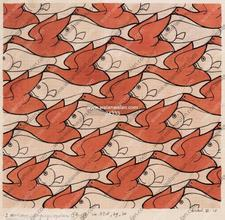Lecture slides     |     Basic equations     |      Text     |     Tools     |     Help

#### Raoult's and Henry's lawsWhat is an ideal solution?## If a solute is surrounded by solvent molecules that are quite different from that molecule then the properties of the solute will be quite different from what they would be in the pure liquid. We must introduce a new theory of non-ideal solutions to explain this difference. The equation that represents the vapor pressure in this case is known as Henry's law. It shows a strong deviation from Raoult's law for dilute solutions

problem solving### Practice as you go...### Problems